• ## 学生成绩管理系统-C语言(附源码)

万次阅读 多人点赞 2019-03-23 14:57:57
#include <stdio.h> #include <string.h> #include <stdlib.h> //结构体 struct score { char snum; char sname; int chinese; int math; int english;... int ra...
这是我初学C时的期末作业，贴出来。
系统功能层次图源代码
//头文件
#include <stdio.h>
#include <string.h>
#include <stdlib.h>
#include <conio.h>

//成绩结构体
struct score
{
char snum;
char sname;
int chinese;
int math;
int english;
int sumcj;//成绩总数
int rank;//排名
};

//函数声明
int xg(int);//修改成绩
void lr(int);//录入成绩
void cx(int);//查询成绩
void xhcx(int);//学号查询
void xmcx(int n);//姓名查询
void allrankcx(int);//排名查询
void xs(int);//成绩显示
void jspm(int);//计算排名
int sc(int);//删除成绩
void bccj(void);//保存成绩并退出

//main函数
int main()
{
int flag,n=0,count=0;//flag标志位,count计数,当前总人数
//-------------判断文件里面有多少条记录--------------------//
FILE *fp;
fp=fopen("cj.txt","a+");
if(fp==NULL)
{
printf("打开文件失败,请重试！\n");
getch();
fclose(fp);
exit(0);
}
int c = 0;
while(!feof(fp))
{
char cc = fgetc(fp);
if(cc==EOF)
{
break;
}
//输出字符
//        putchar(cc);
c++;
}
count = c/14;
//	printf("%d", count);
//	getch();
fclose(fp);
//---------------------------------//
do
{
scanf("%d",&flag);//选择操作
switch(flag)
{
case 1: printf("请输入您要录入的人数：");
scanf("%d",&n);
lr(n);
count += n;break;
case 2: count=sc(count);break;
case 3: xg(count);break;
case 4: cx(count);break;
case 5: jspm(count);break;
case 6: xs(count);break;
case 0: bccj();break;
default:printf("输入错误，请重试！\n");getch();
}
}while(flag!=0);
getch();
return 0;
}

//0显示菜单
{
system("cls");
printf("*----------------------------------------------------*\n");
printf("*               欢迎使用**成绩管理系统               *\n");
printf("*----------------------------------------------------*\n");
printf("*               1： 录入成绩                         *\n");
printf("*               2： 删除成绩                         *\n");
printf("*               3： 修改成绩                         *\n");
printf("*               4： 成绩查询                         *\n");
printf("*               5： 成绩计算并排名                   *\n");
printf("*               6： 显示成绩信息                     *\n");
printf("*               0： 保存信息并退出系统               *\n");
printf("*----------------------------------------------------*\n");
printf("选择操作<0-6>                                         \n");
return;
}

//1录入成绩
void lr(int n)
{
struct score stu;
FILE *fp;
fp=fopen("cj.txt","a+");
if(fp==NULL)
{
printf("打开文件失败,请重试！\n");
getch();
fclose(fp);
exit(0);
}
printf("请输入全部学生的学号，姓名，语文成绩，数学成绩，英语成绩；\n");
int i;
for(i=0;i<n;i++)
{
printf("----第%d个学生----\n", i+1);
printf("学号:");
scanf("%s",&stu[i].snum);

printf("姓名：");
scanf("%s,",&stu[i].sname);

printf("语文成绩：");
scanf("%d",&stu[i].chinese);

printf("数学成绩：");
scanf("%d",&stu[i].math);

printf("英语成绩：");
scanf("%d",&stu[i].english);

stu[i].sumcj=0;
stu[i].rank=0;
//写入数据
fprintf(fp,"%s %s %d %d %d %d %d\n",stu[i].snum,stu[i].sname,stu[i].chinese,stu[i].math,stu[i].english,stu[i].sumcj,stu[i].rank);}
fclose(fp);
printf("添加学生成功,请按任意键返回\n");
getch();
}

//2删除成绩
int sc(int n)
{
struct score stu;
struct score sub;//删除后的结构体数组
char stnum;//学号
int find=0;
printf("请输入你要删除的学生学号:");
scanf("%s", &stnum);
FILE *fp;
fp=fopen("cj.txt","r");
if(fp==NULL)
{
printf("打开文件失败,请联系管理员\n");
getch();
return -1;
}
int c=0;
int index=0;//用于记录目标序号
while(!feof(fp))//不为空
{
fscanf(fp,"%s %s %d %d %d %d %d\n", &stu[c].snum,&stu[c].sname,&stu[c].chinese,&stu[c].math,&stu[c].english,&stu[c].sumcj,&stu[c].rank);
if(strcmp(stnum,stu[c].snum)==0)
{
find = 1;//根据学号查找，找到赋值为1
index = c;//记录编号
}
c++;
}
fclose(fp);
if(n==0)
{
printf("已经没有学生成绩信息了,请按任意键返回\n");
getch();
return n;
}
if(find==0)
{
printf("没有该学生成绩信息,请按任意键返回\n");
getch();
return n;
}
else
{
int i;
//删除
for(i=0;i<c;i++)
{
if(i < index)
{
sub[i] = stu[i];
}
else
{
sub[i] = stu[i+1];
}
}

fp=fopen("cj.txt","w");
//写入数据
for(i=0;i<c-1;i++)
{
fprintf(fp,"%s %s %d %d %d %d %d\n",sub[i].snum,sub[i].sname,sub[i].chinese,sub[i].math,sub[i].english,sub[i].sumcj,sub[i].rank);
}
fclose(fp);
printf("学号为%s的学生成绩删除成功,请按任意键返回\n",stnum);
getch();
return n-1;
}
}

//3修改成绩
int xg(int n)
{
struct score stu;
FILE *fp;
char stnum;
int find=0;
printf("请输入要修改的学生学号:");
scanf("%s",stnum);
fp=fopen("cj.txt","r");
if(fp==NULL)
{
printf("文件打开失败,请联系管理员\n");
getch();
return -1;
}
int c=0;
while(!feof(fp))
{
fscanf(fp,"%s %s %d %d %d %d %d\n",&stu[c].snum,&stu[c].sname,&stu[c].chinese,&stu[c].math,&stu[c].english,&stu[c].sumcj,&stu[c].rank);
if(strcmp(stnum,stu[c].snum)==0)
{
find=1;
//修改操作
printf("找到你要修改成绩的同学,请输入他的成绩:\n");
printf("语文成绩: %d,新成绩为:",stu[c].chinese);
scanf("%d",&stu[c].chinese);
printf("数学成绩: %d,新成绩为:",stu[c].math);
scanf("%d",&stu[c].math);
printf("英语成绩: %d,新成绩为:",stu[c].english);
scanf("%d",&stu[c].english);
}
c++;
}
fclose(fp);
if(find==0)
{
printf("没有找到要删除的学生记录,请按任意键退出\n");
getch();
}
else
{
fp=fopen("cj.txt","w");
int i;
for(i=0;i<n;i++)
{
//更新数据
fprintf(fp,"%s %s %d %d %d %d %d\n",stu[i].snum,stu[i].sname,stu[i].chinese,stu[i].math,stu[i].english,stu[i].sumcj,stu[i].rank);
}
fclose(fp);
printf("学号为%s同学的成绩已经修改成功,请按任意键返回",stnum);
getch();
}
return 1;
}

//4成绩查询
void cx(int n) //n全班人数
{
int k;
do
{
scanf("%d",&k);
switch(k)
{
case 1:xhcx(n);break;//学号查询
case 2:xmcx(n);break;//姓名查询
case 3:allrankcx(n);break;//全班排名查询
case 0:break;
default:printf("输入错误，请重新选择\n");getch();
}
}while(k!=0);
return;
}

//4.0查询菜单
{
system("cls");
printf("*----------------------------------------------------*\n");
printf("*                    成绩查询菜单                    *\n");
printf("*----------------------------------------------------*\n");
printf("*               1： 按学号查询                       *\n");
printf("*               2： 按姓名查询                       *\n");
printf("*               3： 全班排名查询                     *\n");
printf("*               0： 退出查询系统，返回主系统         *\n");
printf("*----------------------------------------------------*\n");
printf("选择操作<0-3>                                         \n");
}

//4.1学号查询
void xhcx(int n)
{
struct score stu;
char snum;
printf("请输入你要查询学生的学号：");
scanf("%s",&snum);
FILE *fp;
fp=fopen("cj.txt ","r");
if(fp==NULL)
{
printf("打开文件失败！\n");
getch();
exit(0);
}
int c = 0;
while(!feof(fp)){
fscanf(fp,"%s %s %d %d %d %d %d\n",&stu[c].snum,&stu[c].sname,&stu[c].chinese,&stu[c].math,&stu[c].english,&stu[c].sumcj,&stu[c].rank);
c++;
}
int i;
for(i=0;i<n;i++)
{
if(strcmp(snum,stu[i].snum)==0)
{
printf("学号\t姓名\t语文\t数学\t英语\t总分\t名次\n");
printf("%s\t%s\t%d\t%d\t%d\t%d\t%d\n",stu[i].snum,stu[i].sname,stu[i].chinese,stu[i].math,stu[i].english,stu[i].sumcj,stu[i].rank);
printf("按任意键返回\n");
getch();
fclose(fp);
return;
}
}
printf("未找到要查询学生的成绩，请重试！\n");
getch();
fclose(fp);
return;
}

//4.2姓名查询
void xmcx(int n)
{
struct score stu;
char sname;
printf("请输入你要查询学生的姓名：");
scanf("%s",&sname);
FILE *fp;
fp=fopen("cj.txt ","r");
if(fp==NULL)
{
printf("打开文件失败！\n");
getch();
exit(0);
}
int c = 0;
while(!feof(fp)){
fscanf(fp,"%s %s %d %d %d %d %d\n\n",&stu[c].snum,&stu[c].sname,&stu[c].chinese,&stu[c].math,&stu[c].english,&stu[c].sumcj,&stu[c].rank);
c++;
}
int i;
for(i=0;i<n;i++)
{
if(strcmp(sname,stu[i].sname)==0)
{
printf("学号\t姓名\t语文\t数学\t英语\t总分\t名次\n");
printf("%s\t%s\t%d\t%d\t%d\t%d\t%d\n",stu[i].snum,stu[i].sname,stu[i].chinese,stu[i].math,stu[i].english,stu[i].sumcj,stu[i].rank);
printf("按任意键返回\n");
getch();
fclose(fp);
return;
}
}
printf("未找到要查询学生的成绩，请重试！\n");
getch();
fclose(fp);
return;
}

//4.3全班排名查询
void allrankcx(int n)
{
struct score stu;
struct score temp;
FILE *fp;
fp=fopen("cj.txt ","r");
if(fp==NULL)
{
printf("打开文件失败！\n");
getch();
exit(0);
}
int c = 0;
while(!feof(fp)){
fscanf(fp,"%s %s %d %d %d %d %d\n",&stu[c].snum,&stu[c].sname,&stu[c].chinese,&stu[c].math,&stu[c].english,&stu[c].sumcj,&stu[c].rank);
c++;
}
int i,j;
for(i=0;i<n;i++)
{
if(stu[i].rank == 0){
printf("请先进行成绩计算进行排名！！\n");
getch();
fclose(fp);
return;
}
stu[i].sumcj = stu[i].chinese + stu[i].math + stu[i].english;
//冒泡排序算法：进行 n-1 轮比较
for(i=0; i<n-1; i++){
//每一轮比较前 n-1-i 个，也就是说，已经排序好的最后 i 个不用比较
for(j=0; j<n-1-i; j++){
if(stu[j].sumcj > stu[j+1].sumcj){
temp = stu[j];
stu[j] = stu[j+1];
stu[j+1] = temp;
}
}
}
printf("学号\t姓名\t语文\t数学\t英语\t总分\t名次\n");
for(i=0;i<n;i++)
{
printf("%s\t%s\t%d\t%d\t%d\t%d\t%d\n",stu[i].snum,stu[i].sname,stu[i].chinese,stu[i].math,stu[i].english,stu[i].sumcj,stu[i].rank);
}
printf("按任意键返回\n");
getch();
fclose(fp);
return;
}
printf("查询失败，请重试！\n");
getch();
fclose(fp);
return;
}

//5计算排名函数
void jspm(int n)
{
struct score stu;
int i,j,temp;
int count;
FILE *fp;
fp=fopen("cj.txt","r");
if(fp==NULL)
{
printf("打开文件失败！\n");
getch();
exit(0);
}
int c = 0;
while(!feof(fp)){
fscanf(fp,"%s %s %d %d %d %d %d\n",&stu[c].snum,&stu[c].sname,&stu[c].chinese,&stu[c].math,&stu[c].english,&stu[c].sumcj,&stu[c].rank);
stu[c].sumcj = stu[c].chinese + stu[c].math + stu[c].english;
c++;
}
fclose(fp);
//计算排名并存储
for(i=0;i<n;i++)
{
temp=1;//第1名
for(j=0;j<n;j++){
if(stu[i].sumcj<stu[j].sumcj) temp++;//如果较小，排名下降1名
}
stu[i].rank=temp;
}
//更新记录
fp=fopen("cj.txt","w");
for(i=0;i<n;i++)
{
fprintf(fp,"%s %s %d %d %d %d %d\n",stu[i].snum,stu[i].sname,stu[i].chinese,stu[i].math,stu[i].english,stu[i].sumcj,stu[i].rank);
}
fclose(fp);
printf("数据重新计算和排名成功,请按任意键返回主菜单。\n");
getch();
return;
}

//6显示成绩信息
void xs(int n)
{
struct score stu;
int i;
printf("成绩信息如下：\n");
FILE *fp;
fp=fopen("cj.txt","r");
if(fp==NULL)
{
printf("打开文件失败,请重试！\n");
getch();
fclose(fp);
exit(0);
}
printf("学号\t姓名\t语文\t数学\t英语\t总分\t名次\n");
int c = 0;
while(!feof(fp))
{
fscanf(fp,"%s %s %d %d %d %d %d\n",&stu[c].snum,&stu[c].sname,&stu[c].chinese,&stu[c].math,&stu[c].english,&stu[c].sumcj,&stu[c].rank);
c++;
}
if(n!=0){//人数不为0
for(i=0;i<c;i++)
{
printf("%s\t%s\t%d\t%d\t%d\t%d\t%d\n",stu[i].snum,stu[i].sname,stu[i].chinese,stu[i].math,stu[i].english,stu[i].sumcj,stu[i].rank);
}
}
fclose(fp);
printf("显示完毕 ,请按任意键返回\n");
getch();
return;
}

//0保存信息并退出系统
void bccj()
{
printf("感谢您的使用，欢迎下次使用!\n");
getch();
return;
}


系统源码文件
链接：https://pan.baidu.com/s/13Zx6RJiUX2e0nxom22kn5A
提取码：5892
开发环境
操作系统：Windows10
开发工具：Dev-C++
PS：限于本人水平，如有不足还请批评、指出。源码？拿走，拿走。

提醒：由于网友反馈，经过自己的调试，发现诸多问题，部分代码不规范，逻辑错误等等，不堪入目，绝不是个良好的程序，但因近期忙于答辩，代码的修改估计在本月月底。在这之前我可能无暇关注博客，对此给大家造成困扰，深感抱歉。——2020.5.18

更新：已对代码进行优化，经过简单调试，没有发现大问题，相信能够满足部分同学的期末作业要求了。源码文件已更新。——2020.5.29

修复BUG：修复删除成绩后，查询成绩信息异常的问题。源码已更新，请重新下载。——2020.6.19
PS：C学得真的是太菜了，请大家多多包涵！！/抱拳/抱拳。。

代码细节优化：考虑到开发工具的兼容问题，已将getch()函数的头文件（conio.h）添加到源代码。函数声明、定义漏写问题（其实是忘了改函数名了）。判断等于NULL报警告？其实该判断是否等于0，大意啊😂。百度云源码再次更新🤣。——2020.7.26
PS：关于使用VS++、VC++的小伙伴，我用Visual Studio 2019调试过了，程序能跑了，如果还是报错不能跑，我也没辙了。。🙃
如果是报的类似于这样的错：error C4996: ‘getch’: The POSIX name for this item is deprecated. Instead, use the ISO C++ conf，可以参考下这个博客：
错误 1 error C4996: getch: The POSIX name for this item is deprecated. Instead, use the ISO C++ conf_故事就酒的博客-CSDN博客


展开全文C语言
• ## 学生成绩管理系统

万次阅读 2019-10-12 20:35:46
输入一个班级的学生的基本信息（包括学号，姓名，性别，5门课程成绩）。 按姓名或者学号查找、增加、修改、删除和保存各个学生的信息。 计算每个学生各门功课总分和平均分，按学号或总分排序输出每个学生的基本...
题目描述
要求使用链表、文件。

输入一个班级的学生的基本信息（包括学号，姓名，性别，5门课程成绩）。

按姓名或者学号查找、增加、修改、删除和保存各个学生的信息。

计算每个学生各门功课总分和平均分，按学号或总分排序输出每个学生的基本信息及总分、平均分和名次。

计算全班各门功课的平均分，显示每门课程中低于平均分的每一个学生的学号，姓名，性别，科目，成绩。

显示每门科目中，成绩在90分以上的学生信息，以及每门科目中不及格的学生信息。

用菜单进行管理

只有正确输入用户名密码才能使用此系统。

所有内容能够保存到文件中。下次进入系统是从文件中读取原有信息。

方案选择
文件法和链表法。因为学生成绩统计需要用文件来储存我们的数据，用户名、密码以及学生成绩，所以我选择使用文件读取或输入有关信息。这样操作起来比较简单，而且逻辑性也比较强。在这里面我还用了些数组，来存放我们读出的数据，后面进行比较的时候也是比较方便的。链表法写起来虽然有点复杂，但是他的效率还是比较高的。

项目功能分析

功能表

角色1:登陆

序号

功能描述

备注

1

登陆系统

2

登陆成功跳转界面

角色2:主菜单

序号

功能描述

备注

1

显示基本信息

2

查找学生信息

3

增加学生信息

4

修改学生信息

5

删除学生信息

6

学生信息排序

7

学生成绩处理

角色3:学生成绩处理

序号

功能描述

备注

1

低于平均分

2

高于90分

3

不及格

项目过程中遇到的问题和解决办法

问题1  使用文件操作数据

问题描述

怎么把文件中的数据读取出来并使用

解决方案

我第一步做的是登陆界面，这时我就需要使用一个文件把数据读取出来。在一开始的时候，自己对文件的掌握程度完全不够。于是就思考如何处理文件中的数据。先要输入自己的账号和密码，第二就是把输入的东西和文件里本有的数据进行比较，于是我就用了fscanf 这个函数，把文件里的数据读取出来，如果相同就登陆成功。

解决结果

登陆界面已经可以成功操作了，而且对后面文件里数据的处理也会比较方便。

问题2 删除

问题描述

怎么将文件里的一串数据进行删除

解决方案

我想开始想的实施用链表，后面在网上看到一些与数据结构有关的东西，建立、初始化、删除、插入等12个基本操作，但是他与我前面写的代码不太吻合。于是按照自己的方法想，后面觉得文件还是可以操作的，我们可以使用“w”打开只写文件，若文件存在则长度清为0，及文件内容和会消失，重新写一份新的文件。先把文件里的数据放入数组里，然后进行比较，若与需要删除的学生姓名或学号不同，则即将他写入文件，大功告成。

解决结果

文件里一连串的数据能够删除了。

个人心得总结
工程时间分为了2各部分，第一部分是对模块化的使用，让我们清楚的了解到使用模块化操作的简便性。在第一部分完成后，我们进行第二部分的工作，自己独立完成一个项目。我选择的是学生成绩统计管理，以应用教材所学的C语言知识为主，综合课本知识完成一个接近实际需要的应用。我觉得它比较适合我目前的情况。在开始这个项目后，我就在构思，我到底需要一个呈现一个怎样的界面，能够清晰明白地造作好系统。在处理这些问题前，我先是对文件的运用做一个基本的掌握，方便后面的使用。

在做这些东西的时候，一定要有自己的构思和安排，想好后一定要写出自己的工作分解和时间节点，那么就可以清晰的按照自己的思路高效率地走下去。我把自己的工作分成了三个部分，主界面、模块化的处理、数据处理。主界面包括登陆和主菜单，我先做的第一部就是登陆，我想它就类似于我们学校网站的学生登陆界面一样，后台需要有存放账户和密码的地方，我把账户和密码都放进一个文件里，然后读取出来，后面很多基本上也是这一个操作。登陆成功后也有一个提示界面，未成功也会有4次重新登陆。第二部分我就开始做显示学生基本信息、学生信息查找、学生信息增加、学生信息修改、学生信息删除这几个部分，学生信息查找有细划分为按姓名查找和按学号查找。第三部分呢，我就开始做数据的排序和处理，感觉到这里已经是非常轻松了。这个项目里我是根据自己要做的操作，模块化的方方式进行处理的，每个模块都写完后，再把它们结合在一起。等我写完所有的模块，总合最后的主函数也是很简单了。

我觉得里面比较麻烦的就是要设计自己的排版，工作量不算大，但就是考虑美观性，一点一点地去修改也是挺麻烦的，但是要做就一定要做的好看，让自己感官上比较舒服。我在构思如何书写自己的程序时，有时按照自己的逻辑发现可能这条路是走不通的，有的换一种方法去思考，在这个过程中，我也有和我的同学交流，有时发现与他们交流一些问题，自己会收获很多，而且，每个人争对同一个问题都有不同的解决方案，有时也会给自己一种茅塞顿开的感觉。

源代码

{

FILE *fp;

int num=1,flag=0;

system("mode con cols=60 lines=20");//改变界面大小

system ("color F9");//白底蓝字

printf("\n\n\n\n       --------------学生成绩管理系统--------------\n\n\n\n");

printf("             账号:");

printf("\n\n");

printf("             密码:");

gotoxy(19,9);

scanf("%s",name);//输入账号

getchar();

gotoxy(19,11);

getchar();

fp=fopen("user.txt","r");//只读的方式打开文件

{

{

flag=1;//相同返回1

break;

}

}

if(flag==1)//相同返回1

{

return 1;

}

else//不同返回0

{

while(num<4)//循环共5次

{

printf("\n\n\n账号或密码错误！你还有%d次输入得机会。",(4-num));

num++;

Sleep(2000);

system("cls");

printf("\n\n\n\n       --------------学生成绩管理系统--------------\n\n\n\n");

printf("             账号:");

printf("\n\n");

printf("             密码:");

gotoxy(19,9);scanf("%s",name);

getchar();

gotoxy(19,11);

getchar();

rewind(fp);

{

{

flag=1;

break;

}

}

if(flag==1)

{

return 1;

}

}

return 0;

}

void seach()//查找

{

int c;

system("color F9");

system("mode con cols=60 lines=20");

while(1)

{

printf("\n\n\n       --------------学生信息查找--------------\n\n");

printf("                    按姓名查找\n");

printf("                    按学号查找\n\n");

printf("       ----------------------------------------\n\n");

printf("                    请输入以上编号：");

scanf("%d",&c);

getchar();

if(c==1)//按姓名查找

{

seach_name();

break;

}

else if(c==2)//按学号查找

{

seach_num();

break;

}

}

}

{

FILE *fp;

int flag=1;

system("cls");

system("color F9");

system("mode con cols=90 lines=30");

printf("\n\n\n                      ------------【增加学生信息】------------\n\n\n");

if((fp=fopen("student.txt","a"))==NULL)//向文件尾部追加数据

{

printf("文件打开失败!\n");

flag=0;

}

if(flag==1)//成功打开文件

{

printf("           请按以下顺序输入学生信息（学号 姓名 性别 语文 数学 英语 物理 体育）：\n\n");

gotoxy(12,9);

scanf("%s %s %s %f %f %f %f %f",stu.num,stu.name,stu.sex,&stu.yw,&stu.sx,&stu.yy,&stu.wl,&stu.ty);//输入

getchar();

if(fprintf(fp,"%s %s %s %5.1f %5.1f %5.1f %5.1f %5.1f\n",stu.num,stu.name,stu.sex,stu.yw,stu.sx,stu.yy,stu.wl,stu.ty)==EOF)//向文件写入数据结束

{

printf("学生信息增加失败！\n\n");

flag=0;//失败返回0

}

if(flag==1)//增加成功

{

printf("           学生信息增加成功！\n\n");

printf("           你输入得学生信息为：\n           %s %s %s %5.1f %5.1f %5.1f %5.1f %5.1f",stu.num,stu.name,stu.sex,stu.yw,stu.sx,stu.yy,stu.wl,stu.ty);

}

fclose(fp);

}

}

void change()//修改

{

struct stu student;//定义数组存放修改的数据

char Scanf;

FILE *fp1,*fp2;

int flag=0,i=0,j=0,f,mark;

system("cls");

system("color F9");

system("mode con cols=90 lines=30");

printf("\n                       --------------修改学生信息--------------\n");

printf("                       请输入需要修改的学生的【姓名】或【学号】：");

scanf("%s",Scanf);

getchar();

if((fp1=fopen("student.txt","r"))==NULL)//只读的方式打开文件

{

printf("学生基本信息读取失败！");

}

printf("            --------------------------------------------------------------\n");

printf("             学    号    姓  名   性别   语文   数学   英语   物理   体育\n");

while(fscanf(fp1,"%s %s %s %f %f %f %f %f",stu.num,stu.name,stu.sex,&stu.yw,&stu.sx,&stu.yy,&stu.wl,&stu.ty)!=EOF)//从文件中读出数据

{

if(strcmp(Scanf,stu.name)==0 || strcmp(Scanf,stu.num)==0)//比较

{

printf("            --------------------------------------------------------------\n");

printf("             %-12s %-8s %-3s   %-6.1f %-6.1f %-6.1f %-6.1f %-6.1f\n",stu.num,stu.name,stu.sex,stu.yw,stu.sx,stu.yy,stu.wl,stu.ty);

flag=1;

mark=j;

}

strcpy(student[j].num,stu.num);

strcpy(student[j].name,stu.name);

strcpy(student[j].sex,stu.sex);

student[j].yw=stu.yw;

student[j].sx=stu.sx;

student[j].yy=stu.yy;

student[j].wl=stu.wl;

student[j].ty=stu.ty;

j++;

}

printf("            --------------------------------------------------------------\n");

fclose(fp1);

printf("\n                              请输入需要更改的项目：");

scanf("%s",Scanf);

getchar();

if(flag==0)

{

printf("                           你输入的项目错误！\n\n");

}

else

{

if(strcmp(Scanf,"学号")==0)

f=1;

else if(strcmp(Scanf,"姓名")==0)

f=2;

else if(strcmp(Scanf,"性别")==0)

f=3;

else if(strcmp(Scanf,"语文")==0)

f=4;

else if(strcmp(Scanf,"数学")==0)

f=5;

else if(strcmp(Scanf,"英语")==0)

f=6;

else if(strcmp(Scanf,"物理")==0)

f=7;

else if(strcmp(Scanf,"体育")==0)

f=8;

switch(f)

{

case 1:{

fp2=fopen("student.txt","w");

printf("                             将学号【%s】修改为：",student[mark].num);

scanf("%s",Scanf);

getchar();

strcpy(student[mark].num,Scanf);

for(i=0;i<j;i++)

{

fprintf(fp2,"%s %s %s %5.1f %5.1f %5.1f %5.1f %5.1f\n",student[i].num,student[i].name,student[i].sex,student[i].yw,student[i].sx,student[i].yy,student[i].wl,student[i].ty);

}

printf("\n                                     修改成功！\n");

fclose(fp2);

};break;

case 2:{

fp2=fopen("student.txt","w");

printf("                             将姓名【%s】修改为：",student[mark].name);

scanf("%s",Scanf);

getchar();

strcpy(student[mark].name,Scanf);

for(i=0;i<j;i++)

{

fprintf(fp2,"%s %s %s %5.1f %5.1f %5.1f %5.1f %5.1f\n",student[i].num,student[i].name,student[i].sex,student[i].yw,student[i].sx,student[i].yy,student[i].wl,student[i].ty);

}

printf("\n                                     修改成功！\n");

fclose(fp2);

};break;

case 3:{

fp2=fopen("student.txt","w");

printf("                             将性别【%s】修改为：",student[mark].sex);

scanf("%s",Scanf);

getchar();

strcpy(student[mark].sex,Scanf);

for(i=0;i<j;i++)

{

fprintf(fp2,"%s %s %s %5.1f %5.1f %5.1f %5.1f %5.1f\n",student[i].num,student[i].name,student[i].sex,student[i].yw,student[i].sx,student[i].yy,student[i].wl,student[i].ty);

}

printf("\n                                     修改成功！\n");

fclose(fp2);

};break;

case 4:{

fp2=fopen("student.txt","w");

printf("                             将语文成绩【%.1f】修改为：",student[mark].yw);

scanf("%s",Scanf);

getchar();

student[mark].yw=atof(Scanf);

for(i=0;i<j;i++)

{

fprintf(fp2,"%s %s %s %5.1f %5.1f %5.1f %5.1f %5.1f\n",student[i].num,student[i].name,student[i].sex,student[i].yw,student[i].sx,student[i].yy,student[i].wl,student[i].ty);

}

printf("\n                                     修改成功！\n");

fclose(fp2);

};break;

case 5:{

fp2=fopen("student.txt","w");

printf("                             将数学成绩【%.1f】修改为：",student[mark].sx);

scanf("%s",Scanf);

getchar();

student[mark].sx=atof(Scanf);

for(i=0;i<j;i++)

{

fprintf(fp2,"%s %s %s %5.1f %5.1f %5.1f %5.1f %5.1f\n",student[i].num,student[i].name,student[i].sex,student[i].yw,student[i].sx,student[i].yy,student[i].wl,student[i].ty);

}

printf("\n                                     修改成功！\n");

fclose(fp2);

};break;

case 6:{

fp2=fopen("student.txt","w");

printf("                             将英语成绩【%.1f】修改为：",student[mark].yy);

scanf("%s",Scanf);

getchar();

student[mark].yy=atof(Scanf);

for(i=0;i<j;i++)

{

fprintf(fp2,"%s %s %s %5.1f %5.1f %5.1f %5.1f %5.1f\n",student[i].num,student[i].name,student[i].sex,student[i].yw,student[i].sx,student[i].yy,student[i].wl,student[i].ty);

}

printf("\n                                     修改成功！\n");

fclose(fp2);

};break;

case 7:{

fp2=fopen("student.txt","w");

printf("                             将物理成绩【%.1f】修改为：",student[mark].wl);

scanf("%s",Scanf);

getchar();

student[mark].wl=atof(Scanf);

for(i=0;i<j;i++)

{

fprintf(fp2,"%s %s %s %5.1f %5.1f %5.1f %5.1f %5.1f\n",student[i].num,student[i].name,student[i].sex,student[i].yw,student[i].sx,student[i].yy,student[i].wl,student[i].ty);

}

printf("\n                                     修改成功！\n");

fclose(fp2);

};break;

case 8:{

fp2=fopen("student.txt","w");

printf("                             将体育成绩【%.1f】修改为：",student[mark].ty);

scanf("%s",Scanf);

getchar();

student[mark].ty=atof(Scanf);

for(i=0;i<j;i++)

{

fprintf(fp2,"%s %s %s %5.1f %5.1f %5.1f %5.1f %5.1f\n",student[i].num,student[i].name,student[i].sex,student[i].yw,student[i].sx,student[i].yy,student[i].wl,student[i].ty);

}

printf("\n                                     修改成功！\n");

fclose(fp2);

};break;

}

}

}

void Delete()//删除

{

struct stu aa;

FILE *fp1,*fp2;

int i=0,j,flag=0,f;

char Scanf;

system("cls");

system("color F9");

system("mode con cols=90 lines=30");

printf("\n                       --------------删除学生信息--------------\n");

printf("                       请输入需要删除的学生的【姓名】或【学号】：");

scanf("%s",Scanf);

getchar();

printf("            --------------------------------------------------------------\n");

printf("             学    号    姓  名   性别   语文   数学   英语   物理   体育\n");

if((fp1=fopen("student.txt","r"))==NULL)//用只读的方式打开文件

{

printf("学生基本信息读取失败！");

}

while(fscanf(fp1,"%s %s %s %f %f %f %f %f",aa[i].num,aa[i].name,aa[i].sex,&aa[i].yw,&aa[i].sx,&aa[i].yy,&aa[i].wl,&aa[i].ty)!=EOF)

{

if(strcmp(Scanf,aa[i].num)==0 || strcmp(Scanf,aa[i].name)==0)//比较

{

printf("            --------------------------------------------------------------\n");

printf("             %-12s %-10s %-3s %-6.1f %-6.1f %-6.1f %-6.1f %-6.1f\n",aa[i].num,aa[i].name,aa[i].sex,aa[i].yw,aa[i].sx,aa[i].yy,aa[i].wl,aa[i].ty);

flag=1;//找到返回1

}

i++;

}

printf("            --------------------------------------------------------------\n");

fclose(fp1);

if(flag==0)

{

printf("\n                      没有找到姓名或学号为【%s】的学生!\n",Scanf);

}

else

{

printf("\n                        确认删除输入【1】,放弃删除输入【0】：");

scanf("%d",&f);

getchar();

if(f==1)

{

fp2=fopen("student.txt","w");// 用只写的方式打开文件

for(j=0;j<i;j++)

{

if(strcmp(Scanf,aa[j].num)!=0 && strcmp(Scanf,aa[j].name)!=0)//重新写入其他人的数据放入文件

{

fprintf(fp1,"%s %s %s %5.1f %5.1f %5.1f %5.1f %5.1f\n",aa[j].num,aa[j].name,aa[j].sex,aa[j].yw,aa[j].sx,aa[j].yy,aa[j].wl,aa[j].ty);

}

}

printf("\n                                 删除成功！\n\n");

}

else

{

printf("\n                      你已放弃删除该学生的成绩信息！\n");

}

}

fclose(fp2);

}

备注：自己的作业，想保存一下，没别的意思，写的也很丑。


展开全文• 学生成绩管理系统 1. 功能模块与界面 （1）教师，学生登录 （2）学生显示自己的全部信息 （3）教师选择功能：课程管理，成绩录入，成绩查询，成绩修改，成绩删除，学生管理。 （4）课程管理中可以添加，修改，...
• 学生成绩管理系统 开发语言：C语言 开发工具：Visual Studio 2019 开发时间：2019.4.14 开发者：summer @一、系统使用展示 @二、系统功能 @三、菜单 @四、录入学生信息 @五、打印学生信息 @六、保存学生信息 @七、...
学生成绩管理系统
开发语言：C语言
开发工具：Visual Studio 2019
开发时间：2019.4.14
开发者：summer
CSDN源码下载：C语言开发简单的学生成绩管理系统
Github项目源码下载：C语言开发简单的学生成绩管理系统
百度网盘源码下载：链接：https://pan.baidu.com/s/1nF53PtRtbuFCNvG7hfylPw 提取码：ar5g
@一、系统使用展示
@二、系统功能
@三、菜单
@四、录入学生信息
@五、打印学生信息
@六、保存学生信息
@七、读取学生信息
@八、统计所有学生人数
@九、查找学生信息
@十、修改学生信息
@十一、删除学生信息
@十二、退出系统
@十三、出错
一、系统使用展示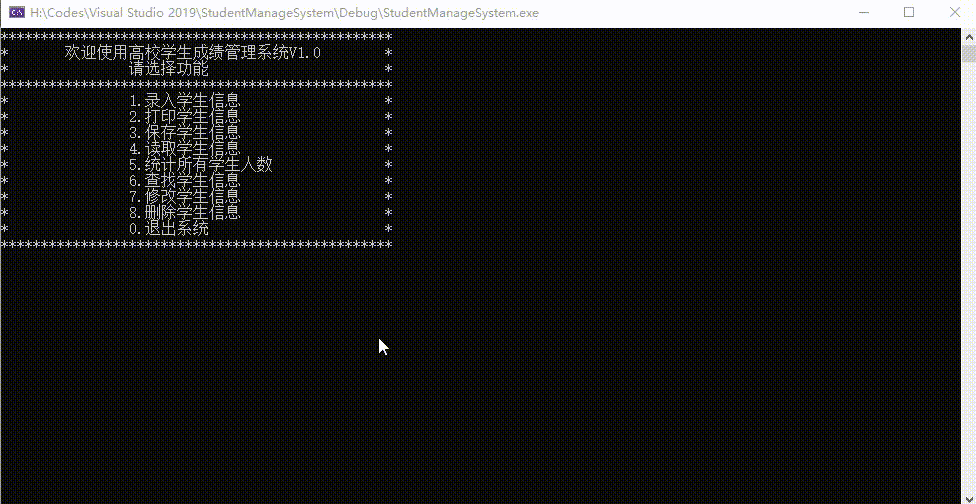二、系统功能

系统功能介绍

1.录入学生信息：用户可以自由输入学生信息到系统中。
2.打印学生信息：格式化展示系统中的学生信息。
3.保存学生信息：将系统中的学生信息保存到本地文档。
4.读取学生信息：读取本地文档中的学生信息并显示。
5.统计所有学生人数
6.查找学生信息：根据用户给定的信息（学号）在系统中查找该学生的信息
7.修改学生信息
8.删除学生信息
0.退出系统


系统main函数，while循环在外，系统程序能够持续运行，switch判断语句进行功能选择与函数切换。

int main() {
while (1)
{
//打印菜单
//读取一个字符 整数,字符在内存中映射为ASCII码
char ch = _getch();

switch (ch)
{
case '1'://1.录入学生信息
InputStudent();
break;
case '2'://2.打印学生信息
PrintStudent();
break;
case '3'://3.保存学生信息
SaveStudent();
break;
case '4'://4.读取学生信息
break;
case '5'://5.统计所有学生人数
CountStudent();
break;
case '6'://6.查找学生信息
FindStudent();
break;
case '7'://7.修改学生信息
ChangeStudent();
break;
case '8'://8.删除学生信息
DeleteStudent();
break;
case '0'://0.退出系统
return 0;
break;
default:
printf("输入有误，没有该功能\n\n");
system("pause");  //暂停
system("cls");    //清屏
break;
}
}

return 0;
}

三、菜单

菜单显示

//菜单
printf("*************************************************\n");
printf("*\t欢迎使用高校学生成绩管理系统V1.0\t*\n");
printf("*\t\t请选择功能\t\t\t*\n");
printf("*************************************************\n");
printf("*\t\t1.录入学生信息\t\t\t*\n");
printf("*\t\t2.打印学生信息\t\t\t*\n");
printf("*\t\t3.保存学生信息\t\t\t*\n");
printf("*\t\t4.读取学生信息\t\t\t*\n");
printf("*\t\t5.统计所有学生人数\t\t*\n");
printf("*\t\t6.查找学生信息\t\t\t*\n");
printf("*\t\t7.修改学生信息\t\t\t*\n");
printf("*\t\t8.删除学生信息\t\t\t*\n");
printf("*\t\t0.退出系统\t\t\t*\n");
printf("*************************************************\n");
}


打印菜单

scanf()、getchar()输入后均需要按enter，而getch()不用
	//打印菜单
//读取一个字符 整数,字符在内存中映射为ASCII码
char ch = _getch();

四、录入学生信息

定义学生结构体、链表节点结构体

//定义一个学生
typedef struct tagStudent {
char szName;	//姓名
char szSex;		//性别
int  nAge;			//年龄
int  nStuNo;		//学号
int  nScore;		//成绩
}Student;

//链表
//节点
typedef struct tagNode
{
Student stu;			//学生信息
struct tagNode* pNext;	//指向下一个节点
}Node;

//创建头节点


利用学生结构体，以及链表操作输入学生信息

//1.录入学生信息
void InputStudent() {
//创建一个人，在堆中分配内存
Node* pNewNode = (Node*)malloc(sizeof(Node));
//指针下一个指向空
pNewNode->pNext = NULL;

//查找链表的尾结点
while (g_pHead != NULL && p->pNext != NULL)
{
p = p->pNext;
}

//把节点插到链表的尾节点
}
else {
p->pNext = pNewNode;
}

//录入学生信息
printf("请输入学生姓名：\n");
scanf_s("%s", pNewNode->stu.szName, sizeof(pNewNode->stu.szName));
printf("请输入性别：\n");
scanf_s("%s", pNewNode->stu.szSex, sizeof(pNewNode->stu.szSex));
printf("请输入学生年龄：\n");
scanf_s("%d", &pNewNode->stu.nAge);
printf("请输入学号：\n");
scanf_s("%d", &pNewNode->stu.nStuNo);
printf("请输入成绩：\n");
scanf_s("%d", &pNewNode->stu.nScore);

printf("学生信息录入成功。\n\n");
system("pause");
system("cls");
}

五、打印学生信息

读取链表结构，输出学生信息。先进行判空处理，如果无学生信息则输出提示信息，反之则正确输出学生信息。

//2.打印学生信息
void PrintStudent() {

system("cls");
//遍历链表
if (p == NULL) {
printf("系统中暂无学生信息，请录入后再来打印查看。\n\n");
}
else {
printf("*********************************************************************************\n");
printf("*\t\t\t欢迎使用高校学生成绩管理系统V1.0\t\t\t*\n");
printf("*********************************************************************************\n");
printf("*\t学号\t*\t姓名\t*\t性别\t*\t年龄\t*\t成绩\t*\n");
printf("*********************************************************************************\n");

while (p != NULL)
{
printf("*\t%d\t*\t%s\t*\t%s\t*\t%d\t*\t%d\t*\n",
p->stu.nStuNo,
p->stu.szName,
p->stu.szSex,
p->stu.nAge,
p->stu.nScore
);

//下一个节点
p = p->pNext;
printf("*********************************************************************************\n");
}
}

system("pause");
system("cls");
}

六、保存学生信息

利用文件对学生信息进行存储

//3.保存学生信息
void SaveStudent() {
//打开文件
FILE* pFile;
pFile = fopen(".\\stuinfo.dat", "w");

if (pFile == NULL) {
printf("打开文件失败。\n");
return;
}

//写入数据
while (p != NULL)
{
fwrite(&p->stu, sizeof(Node), 1, pFile);
p = p->pNext;
}

//关闭文件
fclose(pFile);

printf("数据保存成功。\n");
system("pause");
system("cls");
}


fopen在vs中会报错，解决方式

（1）按照vs的提示，将fopen换成fopen_s
（2）不修改函数，仅仅修改项目的属性。因为fopen_s是一种Microsoft的函数，若纯做Windows开发则直接改为_s也无妨，但是我们尽量还是用标准C++比较好，这样代码有较好的移植性。
具体操作：
右键工程名–>属性–>C/C++–>预处理器–>预处理器定义，编辑右边输入框加入： _CRT_SECURE_NO_WARNINGS
保存（注意用分号隔开）。
七、读取学生信息

打开存储学生信息的文本，单个单词读取，判断非分隔符或者空时则赋值给链表中的节点，读取完毕后，直接调用PrintStudent（）函数进行打印结果。

//4.读取学生信息
system("cls");

//打开文件
FILE* pFile;
pFile = fopen(".\\stuinfo.dat", "r");

if (pFile == NULL) {
printf("打开文件失败。\n");
return;
}

//创建一个人，在堆中分配内存
Node* p = (Node*)malloc(sizeof(Node));
p->pNext = NULL;
//重新建立链表

//逐个单词读入文本内容
char str;
int i = 0;
while (fscanf(pFile, "%s", str) != EOF) {  //读文件
//单词不是*或者空时，进行赋值
if (strcmp(str, "*") && str != NULL) {
switch (i)
{
case 0:
p->stu.nStuNo = atoi(str);
break;
case 1:
strcpy(p->stu.szName, str);
break;
case 2:
strcpy(p->stu.szSex, str);
break;
case 3:
p->stu.nAge = atoi(str);
break;
case 4:
p->stu.nScore = atoi(str);
break;
default:
Node* pNewNode = (Node*)malloc(sizeof(Node));
pNewNode->pNext = NULL;
p->pNext = pNewNode;
p = pNewNode;
p->stu.nStuNo = atoi(str);
i = 0;
break;
}
i++;
}
}
//打印读取结果
PrintStudent();
}


读取文本时，将内容全部赋值给char数组，所有赋值时需特殊处理。

char数组赋值给整数
在<stdlib.h>头文件中有两个函数，int atoi(char *p)和char *itoa(int p),分别将装有整数的char数组转化为整数，和将整数按位数分解依次存入char数组中。

char数组赋值给char数组
用strcpy函数！
原型声明：extern char *strcpy(char *dest,char *src);
头文件：string.h
功能：把src所指由NULL结束的字符串复制到dest所指的数组中。
说明：src和dest所指内存区域不可以重叠且dest必须有足够的空间来容纳src的字符串。
返回指向dest的指针。
注：不能使用memcpy(a, b, strlen(b)),会导致中文乱码。

八、统计所有学生人数

设置计数器，遍历链表

//5.统计所有学生人数
void CountStudent() {
int countStu = 0;

//遍历链表
while (p != NULL)
{
countStu++;
p = p->pNext;
}
printf("学生总人数：%d\n\n", countStu);

system("pause");
system("cls");
}

九、查找学生信息

本次查找以学号为示例，改用姓名等信息查询流程基本一致。
遍历链表，如果有符合信息的学生则打印，反之则继续下一个节点，若系统中无该学生信息，给予用户无结果提示。

//6.查找学生信息
void FindStudent() {
system("cls");
//以学号为查找示例，其他信息查找流程基本相似
int stuNum;
printf("请输入查找学生学号：");
scanf("%d", &stuNum);
//遍历链表查找，查找到后进行信息显示
//对表头进行展示一次
//记录是否有找到该学号的学生信息
bool isFindStu = false;
while (p != NULL)
{
if (stuNum == p->stu.nStuNo) {
printf("*********************************************************************************\n");
printf("*\t学号\t*\t姓名\t*\t性别\t*\t年龄\t*\t成绩\t*\n");
printf("*********************************************************************************\n");
}
printf("*\t%d\t*\t%s\t*\t%s\t*\t%d\t*\t%d\t*\n",
p->stu.nStuNo,
p->stu.szName,
p->stu.szSex,
p->stu.nAge,
p->stu.nScore
);
isFindStu = true;
printf("*********************************************************************************\n");
}
p = p->pNext;
}

if (!isFindStu) {
printf("学号输入有误，系统中暂无该学生信息。\n\n");
}

system("pause");
system("cls");
}

十、修改学生信息

可参考查找学生信息代码段，在查找后进行修改即可

//7.修改学生信息
void ChangeStudent() {
//以学号为查找示例，其他信息查找流程基本相似
int stuNum;
printf("请输入欲修改学生的学号：");
scanf("%d", &stuNum);
//遍历链表查找，查找到后进行信息显示
//对表头进行展示一次
//记录是否有找到该学号的学生信息
bool isFindStu = false;
while (p != NULL)
{
if (stuNum == p->stu.nStuNo) {
printf("*********************************************************************************\n");
printf("*\t学号\t*\t姓名\t*\t性别\t*\t年龄\t*\t成绩\t*\n");
printf("*********************************************************************************\n");
}
printf("*\t%d\t*\t%s\t*\t%s\t*\t%d\t*\t%d\t*\n",
p->stu.nStuNo,
p->stu.szName,
p->stu.szSex,
p->stu.nAge,
p->stu.nScore
);
//修改学生信息
printf("请输入学生姓名：\n");
scanf_s("%s", p->stu.szName, sizeof(p->stu.szName));
printf("请输入性别：\n");
scanf_s("%s", p->stu.szSex, sizeof(p->stu.szSex));
printf("请输入学生年龄：\n");
scanf_s("%d", &p->stu.nAge);
printf("请输入学号：\n");
scanf_s("%d", &p->stu.nStuNo);
printf("请输入成绩：\n");
scanf_s("%d", &p->stu.nScore);
isFindStu = true;
printf("*********************************************************************************\n");
printf("学生信息修改成功，请注意及时保存。\n\n");
}
p = p->pNext;
}

if (!isFindStu) {
printf("学号输入有误，系统中暂无该学生信息，无法进行修改。\n\n");
}

system("pause");
system("cls");
}

十一、删除学生信息

仍然以查找学生信息操作为基础，以学号为线索，查找到则进行删除，未查找到则进行错误提示，删除时需要区分当前节点是头结点、尾节点或者中间节点，不同节点操作不同，同时该删除仅作用于本次操作，需保存学生信息才能长久生效。

//8.删除学生信息
void DeleteStudent() {
system("cls");
//以学号为查找示例，其他信息查找流程基本相似
int stuNum;
printf("请输入删除学生的学号：");
scanf("%d", &stuNum);
//遍历链表查找，查找到后进行信息显示
//记录前一个节点，删除时方便操作

//对表头进行展示一次
//记录是否有找到该学号的学生信息
bool isFindStu = false;
while (p != NULL)
{
if (stuNum == p->stu.nStuNo) {
printf("*********************************************************************************\n");
printf("*\t学号\t*\t姓名\t*\t性别\t*\t年龄\t*\t成绩\t*\n");
printf("*********************************************************************************\n");
}
printf("*\t%d\t*\t%s\t*\t%s\t*\t%d\t*\t%d\t*\n",
p->stu.nStuNo,
p->stu.szName,
p->stu.szSex,
p->stu.nAge,
p->stu.nScore
);
isFindStu = true;
printf("*********************************************************************************\n");

//删除节点为头节点
}
//删除节点为尾节点
else if (p->pNext == NULL) {
p = beforeNode;
p->pNext = NULL;
}
//删除节点为中间节点
else {
beforeNode->pNext = p->pNext;
}
printf("删除成功，请记得保存。\n\n");
}
beforeNode = p;
p = p->pNext;
}

if (!isFindStu) {
printf("学号输入有误，系统中暂无该学生信息，无法进行删除操作。\n\n");
}

system("pause");
system("cls");
}

十二、退出系统
直接在选择0时，设置return即可。
十三、出错
用户输入有误时，进行提示信息输出即可。
	printf("输入有误，没有该功能\n\n");
system("pause");  //暂停
system("cls");    //清屏



展开全文C语言 链表
• 通过C语言开发一个简单的项目：学生成绩管理系统 1.C语言知识点的综合运用，加深对C语言的理解，学会结构化编程的思想 2.熟悉企业软件开发的基本流程，树立软件工程的思维和概念 3.具备独立开发一个小型甚至中型软件...软件设计 软件设计说明书 软件设计文档 软件设计模式 软件设计原则
• 6.0.8版本升级了以下内容： 1、锁定考试项目以后还能录成绩的BUG 2、写留言版对齐问题 3、任课教师录成绩会显示...（2）五大功能模块：1、学籍管理 2、成绩管理 3、作业管理（美术培训学校专用） 4、学生素质评价php源码
• 1.学生管理系统的实现：在我的程序里，MFC主对话框“学生管理系统”中拥有“保存”，“打开”，“添加”，“删除”，“按高数成绩排序”，“按C++成绩排序”，“修改”，“确定”，“反选”一共9个按钮，以及“学号...
如题
********菜鸡的课设，仅供交流参考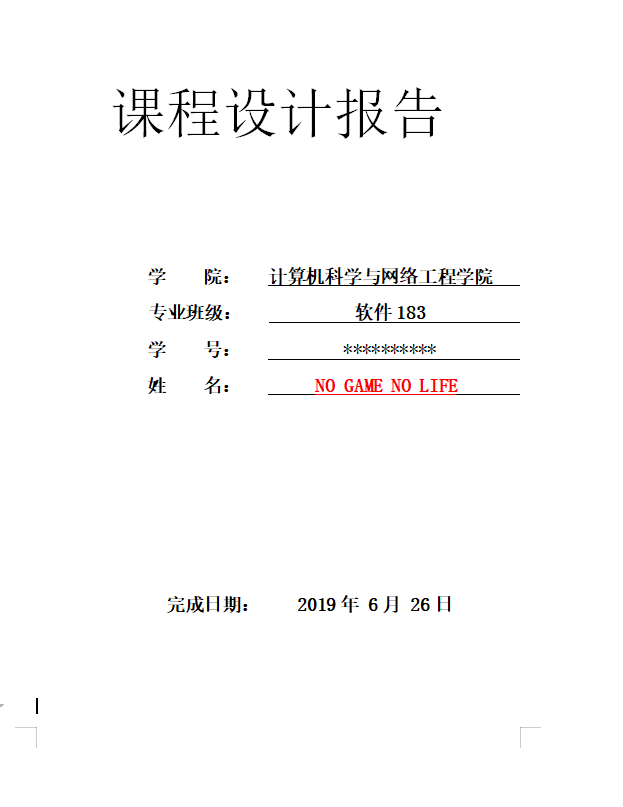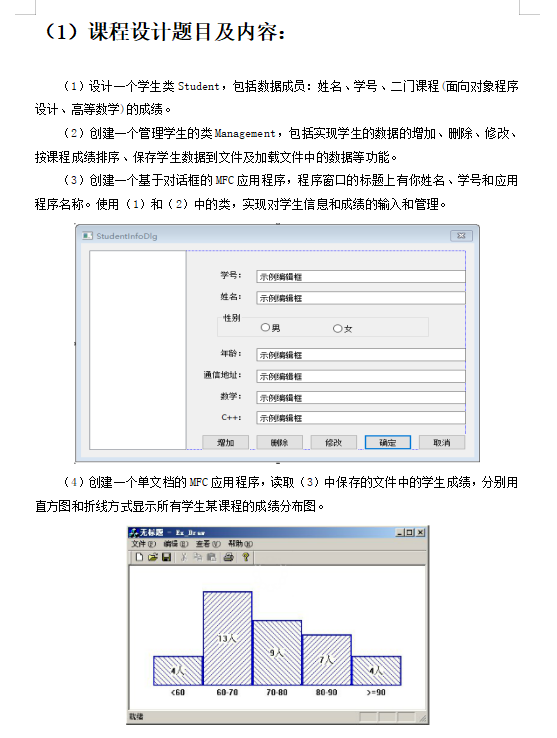（2）程序功能模块的设计：
1.学生管理系统的实现：
在我的程序里，MFC主对话框“学生管理系统”中拥有“保存”，“打开”，“添加”，“删除”，“按高数成绩排序”，“按C++成绩排序”，“修改”，“确定”，“反选”一共9个按钮，以及“学号”，“姓名”，“高数”，“C++”四个编辑框，还有一个list control列表用于显示数据。四个编辑框绑定了4个CString变量，利于直接获取数据，
在students managementDlg.cpp中拥有list control初始化的代码以及8个按钮分别对应实现的代码。在student.h头文件，里面用于存放一个“学生类”，在public中存放学生的姓名，学号，高数成绩，c++成绩，（分CString以及long long）两个类型存储，用一个convert成员函数实现从CString数据转变为long long存储以便实现排序功能。
在mangement.cpp中实现“学生类”以及“管理类”中的函数。
2.数据用直方图以及折线图显示的实现：
在MFC单文档中的View.h的类CView的public添加两个按钮CButton函数以及一个函数Open。在View.cpp中的OnDraw函数里实现的绘图，在类向导中添加一个OnCreate函数，并且实现按钮的显示。最后实现两个按钮的函数（打开“学生管理系统”中保存的save.txt，根据提取save.txt中的数据更改各个分段的高度以显示出直方图以及折现图）。
（3）带有详细注释的自己编写的关键源程序：
1.学生管理系统：
//将四个编辑框分别绑定四个CString变量：Id,name,math,Cjj

1.Student.h头文件：
#include <afxtempl.h>
#define ll long long
using namespace std;
#pragma once

class student
{
public:
//除了name以外所有数据均存一份CString跟一份long long
ll Idq;
ll mathq;
ll Cjjq;
CString name;//姓名
CString Id;//学号
CString math;//高数成绩
CString Cjj;//C++成绩
void convert();//将存储的CString转换成long long
};

2.Manageff.h头文件：
#pragma once
#include "stdafx.h"
#include "Student.h"

class studentmanag
{
//学生类数组容器类
private:
int len;//容器总大小
int count;//容器已存的大小

public:
studentmanag();//构造函数
student * group;//学生数组容器

int getcount();//获取容器已存大小
void del(int x);//从容器中选择删除一个学生
void math_sort();//按高数成绩排序数组
void Cjj_sort();//按C++成绩排序数组
bool getsame(student a);//判断容器中是否有学号相同的人
};

3.Management.cpp：
#include "stdafx.h"
#include "Student.h"
#include "manageff.h"
#include <algorithm>

using namespace std;

void student::convert()
{
//将CString转成long long型存储
Idq = _ttoi(Id);
mathq = _ttoi(math);
Cjjq = _ttoi(Cjj);
return;
}

studentmanag::studentmanag()
{
//初始化
group = new student;
len = 100;
count = 0;
}

int studentmanag::getcount()
{
//获取容器已存大小
return this->count;
}

{
//容器添加操作
count++;
if (count >= len)//如果现有大小等于容器大小，则扩大容器
{
student *p = new student[len + 10];//开辟新容器
len += 10;
for (int i = 0; i < count - 1; i++)//将新容器复制旧容器
{
p[i] = group[i];
}
delete[]group;//删除旧容器的空间
group = p;//绑定新容器
}
group[count - 1] = x;
return;
}

void studentmanag::del(int x)
{
//容器删除操作
count--;
int times = count - x;
while (times--)
{
group[x] = group[x + 1];
x++;
}
return;
}

bool cmp1(student x, student y)
{
//用于排序高数成绩的cmp
if (x.mathq == y.mathq)
{
return x.Cjjq > y.Cjjq;
}
return x.mathq > y.mathq;
}

void studentmanag::math_sort()
{
//按高数成绩排序
sort(group, group + count, cmp1);
return;
}

bool cmp2(student x, student y)
{
//用于排序c++成绩的cmp
if (x.Cjjq == y.Cjjq)
{
return x.mathq > y.mathq;
}
return x.Cjjq > y.Cjjq;
}

void studentmanag::Cjj_sort()
{
//按C++成绩排序
sort(group, group + count, cmp2);
return;
}

bool studentmanag::getsame(student a)
{
//判断容器中是否存在学号相同的人
ll num = a.Idq;
bool flag = 0;
for (int i = 0; i < count; i++)
{
if (this->group[i].Idq == num)
{
flag = 1;
break;
}
}
return flag;
}

4.students managementDlg.cpp中关键源代码：
OnInitDialog函数中添加:
//这是listcontrol的初始化，初始化添加勾选框
m_list.SetExtendedStyle(LVS_EX_FULLROWSELECT|LVS_EX_CHECKBOXES);
m_list.InsertColumn(0, _T("学号"),0,80);
m_list.InsertColumn(1, _T("姓名"),0, 80);
m_list.InsertColumn(2, _T("高数成绩"),0, 99);
m_list.InsertColumn(3, _T("C++成绩"),0, 97);

按钮实现：
在students manageDlg.cpp中添加
studentmanag stdmanag;
void CstudentsmanageDlg::OnBnClickedButton3()
{
//添加按钮

UpdateData(TRUE);
//将编辑框中的数据写入学生类
student a;
a.Cjj = Cjj;
a.Id = Id;
a.math = math;
a.name = name;
a.convert();

if (stdmanag.getsame(a))
{
CString k = "学号重复了！";
MessageBox(k);
//弹出信息框k
}
else
{

//将数据在列表中显示出来
int ncount = m_list.GetItemCount();
m_list.InsertItem(ncount, a.Id);
m_list.SetItemText(ncount, 1, a.name);
m_list.SetItemText(ncount, 2, a.math);
m_list.SetItemText(ncount, 3, a.Cjj);
}
}

void CstudentsmanageDlg::OnBnClickedButton2()
{
//删除按钮

for (int i = 0; i < m_list.GetItemCount(); i++)
{
//判断是否勾选
BOOL check = m_list.GetCheck(i);
if (check)
{
stdmanag.del(i);
//调用删除函数
m_list.DeleteItem(i);
//删除列表中该行数据
i--;
}
}
}

void CstudentsmanageDlg::OnBnClickedButton1()
{
//修改按钮

for (int i = 0; i < m_list.GetItemCount(); i++)
{
BOOL check = m_list.GetCheck(i);
if (check)
{
//如果勾选了该项
UpdateData(TRUE);

student a;
a.Cjj = Cjj;
a.Id = Id;
a.math = math;
a.name = name;
a.convert();
stdmanag.group[i] = a;
//根据编辑框中数据更新容器中学生数据

int ncount = m_list.GetItemCount();
m_list.SetItemText(i, 0, a.Id);
m_list.SetItemText(i, 1, a.name);
m_list.SetItemText(i, 2, a.math);
m_list.SetItemText(i, 3, a.Cjj);
//更新列表数据显示
}
}
}

void CstudentsmanageDlg::OnBnClickedButton4()
{
//按高数成绩排序按钮

int count = stdmanag.getcount();
stdmanag.math_sort();
//调用排序

m_list.DeleteAllItems();
//删除所有列表中所有数据
for (int i = 0; i < count; i++)
{
//容器中所有容器数据添加进列表
m_list.InsertItem(i, stdmanag.group[i].Id);
m_list.SetItemText(i, 1, stdmanag.group[i].name);
m_list.SetItemText(i, 2, stdmanag.group[i].math);
m_list.SetItemText(i, 3, stdmanag.group[i].Cjj);
}
}

void CstudentsmanageDlg::OnBnClickedButton5()
{
//按C++成绩排序按钮

int count = stdmanag.getcount();
stdmanag.Cjj_sort();
//调用排序

m_list.DeleteAllItems();
//删除所有列表中所有数据

for (int i = 0; i < count; i++)
{
//容器中所有容器数据添加进列表
m_list.InsertItem(i, stdmanag.group[i].Id);
m_list.SetItemText(i, 1, stdmanag.group[i].name);
m_list.SetItemText(i, 2, stdmanag.group[i].math);
m_list.SetItemText(i, 3, stdmanag.group[i].Cjj);
}
}

void CstudentsmanageDlg::OnBnClickedButton7()
{
//打开按钮

CFileDialog saveDlg(TRUE, ".txt", "save", OFN_OVERWRITEPROMPT, "文本文档(*.txt)|*.txt|所有文件(*.*)|*.*||", NULL);
//设置保存文件对话框格式

if (saveDlg.DoModal() == IDOK)
{
//如果按了保存

//获取文件路径，并且设置只读
CString path = saveDlg.GetPathName();

CString date;
student ui;
int i = 0, j = 0;

{
//将数据显示在列表中
if (i % 4 == 0)
{
m_list.InsertItem(j, date);
}
else
{
m_list.SetItemText(j, i % 4, date);
}

//将数据添加进容器
if (i % 4 == 0)
{
//第一行为学号
if (j != 0)
{
//除了第一个数据之外，当为4倍数时即说明ui已获取完一个学生数据
//然后添加进容器
ui.convert();
}
ui.Id = date;
}
else if (i % 4 == 1)
{
//第二行为姓名
ui.name = date;
}
else if (i % 4 == 2)
{
//第三行为高数成绩
ui.math = date;
}
else if (i % 4 == 3)
{
//第四行为C++成绩
ui.Cjj = date;
}
i++;
if (i % 4 == 0) j++;
//四个数据一换行
}
//将最后一个数据传进容器
ui.convert();
}
}

void CstudentsmanageDlg::OnBnClickedButton8()
{
//保存按钮

CFileDialog saveDlg(FALSE, ".txt", "save", OFN_OVERWRITEPROMPT, "文本文档(*.txt)|*.txt|所有文件(*.*)|*.*||", NULL);
//设置保存文件对话框格式

if (saveDlg.DoModal() == IDOK)
{
//如果按了保存

CString path = saveDlg.GetPathName();//获取文件路径
CString k = "文件已保存在路径";
CString k2;
k2.Format("%s%s", k, path);//k+k2
MessageBox(k2);
//弹出信息框k2

CStdioFile file(path,CFile::modeWrite|CFile::modeCreate);
//创建或者写入文件

//保存数据
CString cs, hh = "\n";
for (int i = 0; i < m_list.GetItemCount(); i++)
{
//i行
for (int j = 0; j < 4; j++)
{
//j列
cs = m_list.GetItemText(i, j);
file.WriteString(cs);
//写入一个数据
file.WriteString(hh);
//换行
}
}
file.Close();
//关闭文件
}
}

void CstudentsmanageDlg::OnBnClickedButton9()
{
//反选按钮
for (int i = 0; i < m_list.GetItemCount(); i++)
{
m_list.SetCheck(i, !m_list.GetCheck(i));//设置是否勾中
}
}

2.直方图以及折线图的显示：
在Doc.cpp中的OnNewDocument函数中添加以下代码（修改标题）
SetTitle(_T("直方图/折线图实现"));

1.在View.h中的Cview类的public添加以下代码：
afx_msg void OnClickButton1();
afx_msg void OnClickButton2();
//定义按钮1,2
CButton m_button;
CButton m_button1;

2.View.cpp中关键源代码：
int a = 0, b = 0, c = 0, d = 0, e = 0;
//矩阵高度
//在Ondraw函数中添加以下代码：
pDC->SetMapMode(MM_ISOTROPIC);
pDC->SetViewportOrg(200,450);//设置原点
pDC->MoveTo(100, 0);//从100，0画到1300，0
pDC->LineTo(1300, 0);

//设置画笔参数
CBrush brush;
//画笔颜色
brush.CreateHatchBrush(HS_BDIAGONAL, RGB(150, 100, 45));
pDC->SelectObject(brush);

int i = 1;

//绘制矩形,分别为四个点坐标
pDC->Rectangle(200, 0, 400, 50*a);
pDC->Rectangle(400, 0, 600, 50 * b);
pDC->Rectangle(600, 0, 800, 50 * c);
pDC->Rectangle(800, 0, 1000, 50 * d);
pDC->Rectangle(1000, 0, 1200, 50 * e);

//设置分段文本坐标
pDC->TextOut(250, 0, " <60");
pDC->TextOut(450, 0, "60-70");
pDC->TextOut(650, 0, "70-80");
pDC->TextOut(850, 0, "80-90");
pDC->TextOut(1050, 0, "90-100");

//设置文字高度（多少人）
CString y1,y2,y3,y4,y5;
y1.Format("%d", a);
y2.Format("%d", b);
y3.Format("%d", c);
y4.Format("%d", d);
y5.Format("%d", e);

//x轴从250-1050
pDC->MoveTo(250, 50 * a + 100);
pDC->LineTo(450, 50 * b + 100);
pDC->LineTo(650, 50 * c + 100);
pDC->LineTo(850, 50 * d + 100);
pDC->LineTo(1050, 50 * e + 100);

//设置文本位置
if(a!=0)
pDC->TextOut(250, 27 * a, y1 + "人");
if (b != 0)
pDC->TextOut(450, 27 * b, y2 + "人");
if (c != 0)
pDC->TextOut(650, 27 * c, y3 + "人");
if (d != 0)
pDC->TextOut(850, 27 * d, y4 + "人");
if (e != 0)
pDC->TextOut(1050, 27 * e, y5 + "人");
//在类向导中添加处理程序OnCreate
//在View.cpp中OnCreate函数添加以下代码：
m_button.Create("高等数学",//设置按钮1标题
WS_CHILD | WS_VISIBLE | WS_BORDER,

//设置按钮1坐标
CRect(1200, 10, 1360, 60),
this,
IDC_BUTTON1);
m_button.ShowWindow(SW_SHOWNORMAL);

m_button1.Create("C++",//设置按钮2标题
WS_CHILD | WS_VISIBLE | WS_BORDER,

//设置按钮2坐标
CRect(1200, 70, 1360, 120),
this,
IDC_BUTTON2);
m_button1.ShowWindow(SW_SHOWNORMAL);

//两个按钮的函数实现
void CDrawT12View::OnClickButton1()
{
//按钮1实现

//初始化
a = b = c = d = e = 0;

//打开文件并且操作
CFileDialog fdlg(TRUE, ".dat", "save", OFN_OVERWRITEPROMPT, "数据文件(*.txt)|*.txt|所有文件(*.*)|*.*||", NULL);

//如果成功读取文件
if (fdlg.DoModal() == IDOK)
{
CString cs;

int i = 0;
{
//当还能读取到行
if (i % 4 == 2)
{
//如果是高数成绩，则转换为数值再判断大小
long long q = _ttoi(cs);
if (q < 60) a++;
else if (q >= 60 && q <= 70) b++;
else if (q > 70 && q <= 80) c++;
else if (q > 80 && q <= 90) d++;
else if (q > 90) e++;

}
i++;
}
}
InvalidateRect(NULL);
}

void CDrawT12View::OnClickButton2()
{
//按钮2实现

//初始化
a = b = c = d = e = 0;

//打开文件并且操作
CFileDialog fdlg(TRUE, ".dat", "save", OFN_OVERWRITEPROMPT, "数据文件(*.txt)|*.txt|所有文件(*.*)|*.*||", NULL);

//如果成功读取文件
if (fdlg.DoModal() == IDOK)
{
CString cs;

int i = 0;
{
//当还能读取到行
if (i % 4 == 3)
{
//如果是C++成绩，则转换为数值再判断大小
long long q = _ttoi(cs);
if (q < 60) a++;
else if (q >= 60 && q <= 70) b++;
else if (q > 70 && q <= 80) c++;
else if (q > 80 && q <= 90) d++;
else if (q > 90) e++;
}
i++;
}
}
InvalidateRect(NULL);
}

（4）程序运行时的效果图：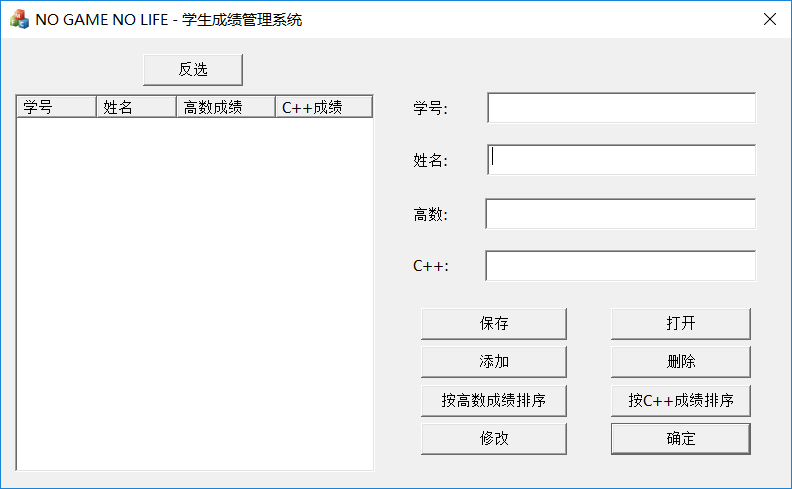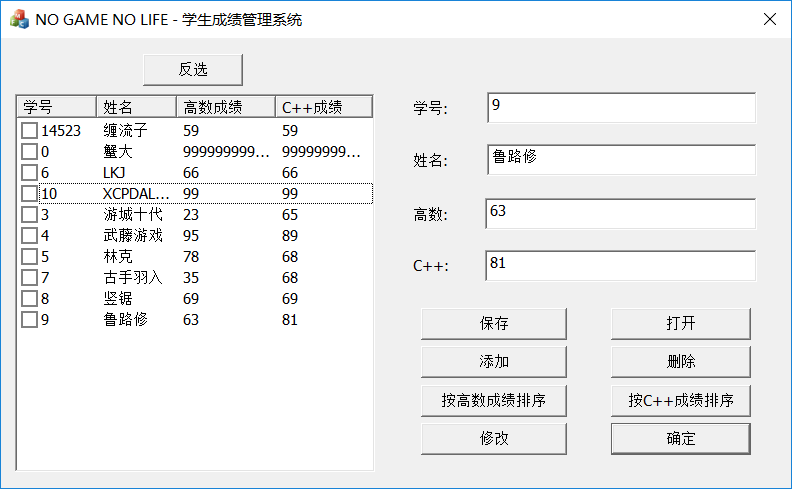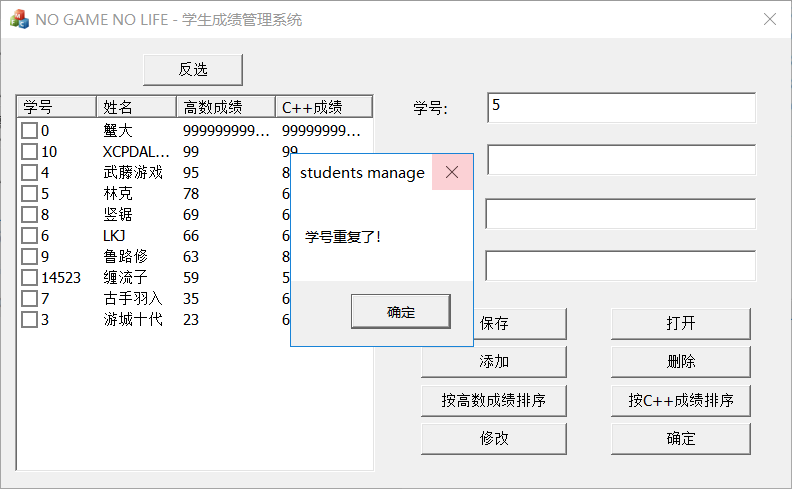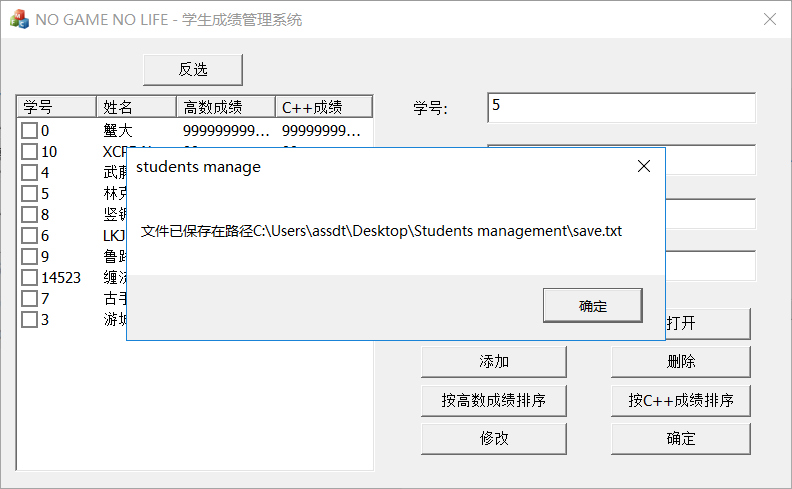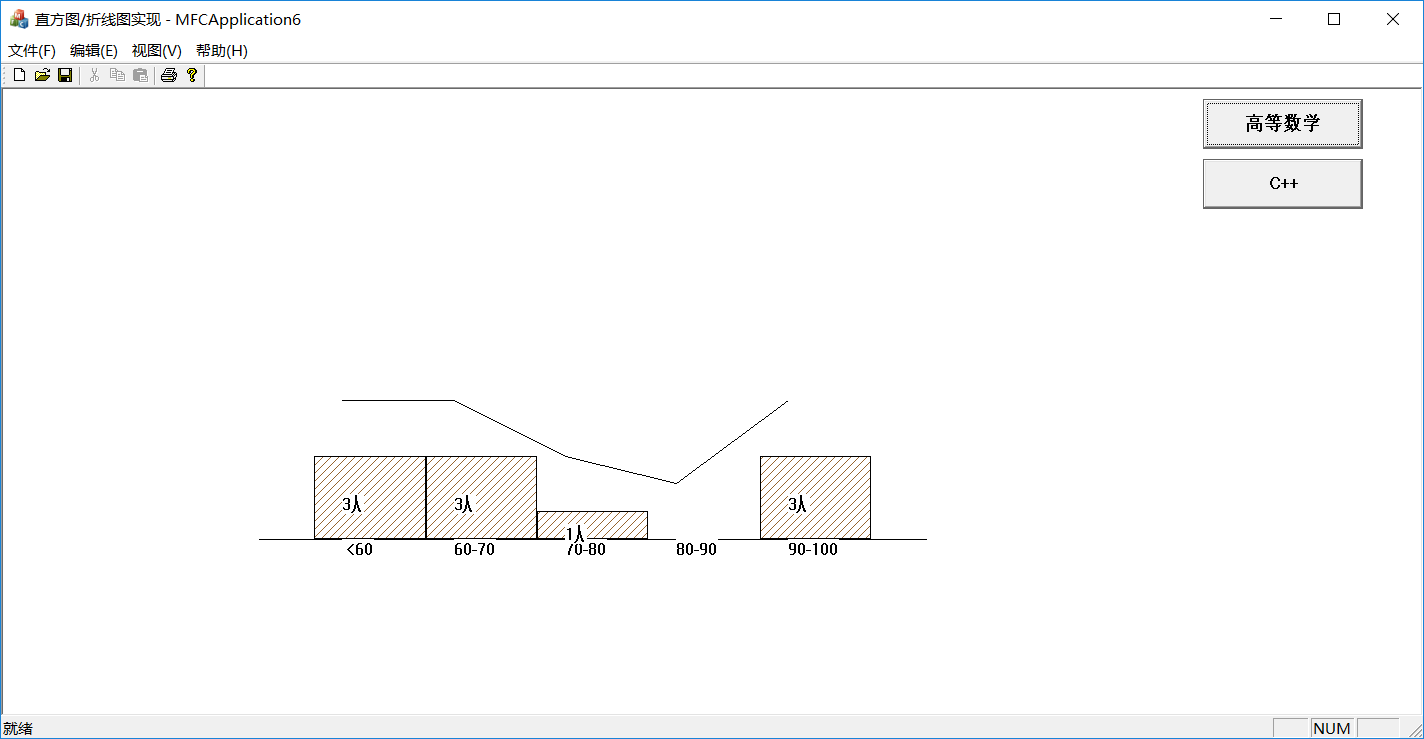（5）.实验结果分析，实验收获和体会：
这次mfc实验是对话框实现以及View单文档视图的综合应用
1.这次实验进行初期，我对MFC中工具箱中控件跟实际映射的变量以及函数应用不太熟练，经过实验，我熟悉了MFC的Dlg界面设计，以及各个控件之间的关联绑定。
2.这次实验需要大量使用MFC中各个控件的自带函数（例如读写txt文件，list control的函数方法等等），需要在网上查找资料，经过实验后，使得我对这些函数的使用更加的熟悉了。
3.经过实验，我巩固了头文件的编写与多cpp的编写形式。
4.在cpp文件运用头文件中书写的类时会出现很多问题：无法识别头文件中的类，将头文件stdafx.h书写在最前面include就解决了问题，具体原因未知。
5.试验初期，我运用了CArray（MFC自带的模板容器）来存储学生类，很快就完成完了要求，但是后来因为老师不允许使用STL容器以及其他容器，所以自己写了一个容器，并且通过自己实现了很多类容器的函数方法，让我更加提升了自己的代码能力。
6.实验要求实现MFC单文档内展示两个不同的直方/折现图，因此需要在单文档视图中添加按钮实现，于是我在网上查找资料学习到了在MFC单文档中安置按钮的方法，以及熟悉了在MFC中CBrush（画笔）的函数方法使用。
7.实现排序功能时，我写了多个cmp用于实现多个排序方法，再用algorithm头文件中的sort函数进行快排，实现便捷并且效率高。
8.在实现直方图/折线图的时候，实现界面的标题是未定义标题，觉得有点不太好看（截图在实验报告不美观），因此在网上查找资料，学会了更改标题的方法。
（6）实验的改进意见和建议：
1.实验要求中没有要求到的，我觉得挺重要的按钮：按学号查找，我的想法是在容器类中添加find函数用于查找参数中输入的int学号，再弹出对话框显示该学号学生的所有信息。
2.在学生管理系统中，虽然实验没有要求，但是我添加了反选的按钮，因为我发现在调试的时候，要一个个勾选删除比较麻烦，所以添加反选按钮实现一键删除功能。
3.我认为判断是否学号重复添加的功能是很需要的，因此我在类容器中写了个查找学号是否重复的函数，用于实现这个功能，若重复添加则弹出“学号重复”信息框。
总结：（之前觉得MFC是个上世纪的产物，觉得没用，课也没听，但是为了写这课程设计，还特地去学，枯辽，但是感觉学会了还行8）


展开全文c++ 可视化
• ## MFC课程设计 --学生成绩管理系统

万次阅读 多人点赞 2018-07-26 21:12:55
MFC课程设计 ,C++课程设计 --学生成绩管理系统 ps:因为课设完成的过程大家都不太一样，以下的代码仅供学习一下在MFC下各个控件的用法，有问题欢迎留言讨论。     实验目的 使用MFC类库编制应用程序：按钮...c
• ## 《C语言编写 学生成绩管理系统》

万次阅读 多人点赞 2012-06-23 12:16:23
/* (程序头部注释开始) ...* 文件名称： 学生成绩管理系统 * 作 者： 刘江波 * 完成日期： 2012 年 6 月 23 日 * 版 本 号： v.623 * 对任务及求解方法的描述部分 * 程序头部的注释结束 */语言 c fp struct float
• ## java学生成绩管理系统

万次阅读 多人点赞 2016-04-12 14:33:51
*@name:java学生成绩管理系统. *@功能：学生相关信息，录入，查询，统计，修改等.... *@PS：图形界面的学生管理系统不要求就不做了. */ import java.util.Scanner; import java.lang.*; import jajava
• ## java学生信息管理系统（附源码）

万次阅读 多人点赞 2016-07-14 16:36:30
一： 介绍 1：实现登录界面 2：实现主界面 有两种操作 一种是按钮操作 另外一种是SQL语句。 ... 3：连接SQL server 2008 数据库,后续我更新了，加了一个MySql 版本。在我的下载链接里面都有，里面也有使用说明...连接SQL数据库
• ## java学生成绩管理系统界面设计

万次阅读 多人点赞 2018-06-29 10:35:40
关于学生成绩管理系统的界面设计:代码如下 1import&nbsp;javax.swing.*; 2import&nbsp;java.awt.*; 3import&nbsp;java.awt.event.*; 4import&nbsp;com.example.SqlHelper; 5class&nbsp;...
• ## Java学生成绩管理系统

万次阅读 多人点赞 2018-01-13 00:42:37
教学管理系统很适合初学者对于所学语言的练习。本文是javaSE 中用文件流写的，这个也可以用数据库写。分析这个项目有 1.学生 2.老师 3.教务人员 4.管理员四个角色分别担任不同的任务。1.学生 有属性 id, 密码，性别...文件读写
• ## Java学生成绩管理系统

万次阅读 多人点赞 2019-09-21 09:32:45
目录 引言 开发环境 所用技术 项目目录 数据库设计 ...这是当时刚学完SpringMVC、Spring-Data-Jpa和Hibernate后台框架写的一个项目。一是月考核的项目，二是刚学完这些框架，需要练习一下，总结一下。...
• ## JavaWeb学生成绩管理系统

万次阅读 多人点赞 2018-07-06 21:12:05
基于JavaWeb开发的学生成绩，使用MyEclipse2014开发，连接MySQL数据库，存储学生的身份信息、成绩，管理员的身份信息，课程信息。功能说明：学生注册。学生身份:跳转到学生登录界面，点击查询成绩按钮，以表格的形式...JSP JavaWeb
• JAVA学生成绩管理系统（附源码 毕业设计 课程设计） 可直接运行。做毕业设计、课程设计或者想研究下技术的可以下载学习。需要更多资源的可以关注我。
• java 学生成绩管理系统 源代码 import java.applet.Applet; import java.awt.*; import java.awt.event.*; import java.util.*; import java.lang.*; public class Students.java extends Applet implements ...
• java写的学生成绩管理系统，数据库用的是SQL Sever,里面包含代码和过程分析，还有界面 用java写的学生成绩管理系统，数据库用的是SQL Sever,里面包含代码和过程分析，还有界面
• Grade类：用来存储学生的信息 import java.io.Serializable; class Grade implements Serializable{ private int id; private String name; private double math; private double english; private double ...
• 简单java学生成绩管理系统，无UI界面，连接SQL数据库 import java.sql.*; import java.util.Scanner; public class StudentManagement { //取得数据库的连接 String driverName=&amp;quot;...新手 连接数据库string import null file
• 学生成绩管理系统（单链表+java的GUI） 说明： 1.我所说的保存到数据库并没有保存到数据库。保存数据的生命周期仅仅是运行到关闭之间时间。 2.用的是单链表，所涉及的功能有：增加，删除，修改，查看。 3.无论你之前...
• 一、题目: 用文件实现的学生成绩管理系统 二、目的 学生通过本次实验编程实现一个班级学生成绩的管理，使学生了解文件的主要操作（创建、读、写、增加和删除记录等）。 三、内容和要求 1、编写一个学生成绩管理的...
• ## C语言学生成绩管理系统源代码

万次阅读 多人点赞 2018-03-21 20:27:20
大学C语言实训课，C语言学生成绩管理系统。 #include<stdio.h> #include<string.h> #include<math.h> struct student { int num; char name; float pingshi; float shiyan; ...C语言成绩管理 C语言管理系统 管理系统
• 这是java版的学生成绩管理系统，采用ＧＵＩ界面，ｓｗｉｎｇ组件进行设计，基本功能是对保存在数据库中学生成绩进行查询，添加，删除，修改，排序功能，较为简单。程序比较长，所以没怎么写注释，如有疑问可以给我发...
• java实现学生成绩管理系统Java完成算法设计和程序设计并上机调试通过。程序添加适当的注释，程序的书写要采用缩进格式。程序具在一定的健壮性，即当输入数据非法时，程序也能适当地做出反应，如插入删除时指定的...
• import java.awt.*; import java.awt.event.*; import javax.swing.*; import javax.swing.JFrame; import javax.swing.JLabel; import javax.swing.JPanel; import javax.swing.JButton; import javax.swing.JTex.....MySQL
• ## JAVA 实现简单的学生成绩管理系统

万次阅读 多人点赞 2018-04-15 10:27:23
2．掌握简单的信息管理系统的设计与实现。二、实验环境实验建议在安装了以下软件的计算机上完成：1. Windows xp/win7/win8/win10操作系统2. JDK 1.6以上版本3. Eclipse或NetBeans IDE或EditPlus或其它开发工具三......